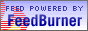## The kalam argument

First of all, the assumption is that the universe has to be explained (Russell would say "It's just there").

There are three points to the kalam argument:
1. The universe had a beginning
2. The universe was caused
3. This cause was personal
With regard to the first point, it is important to make a distinction between an actual infinite and a potential infinite.

We call actual infinite a timeless totality which neither increases nor decreases in the number of elements it contains. As is clear from the theory of infinite sets, a subset of an infinite set contains an infinite number of elements (i.e. this set can be put in a 1-1 correspondence with the actual infinite).

We call potential infinite a series that increases the number of its elements by addition. In this case obviously there is no 1-1 correpondence between a subset and the potential infinite.

Having said that, it seems that point 1 is warranted by at least the following arguments:
• the fact that an actual infinite cannot exist in the real world. IOW, it is not possible to instantiate an actual infinite in time. In time is the keyword here. Indeed: a dimention of the universe is time; if an actual infinite existed, then one can conceive the universe as beginningless; then an infinite numbers of events would have occurred in time. But if some of these were collected into a set, this set would have to have an infinite number of members. But it does not seem possible that this occurs in the real world. From the fact that we have a mathematic theory of infinite numbers does not follow that this theory applies to the real world (similarly, we can think of many geometries of the space, but not all of them have a real correspondence in the world).
• the medieval argument about the impossibility of traversing an actual infinite, assuming (against the point above) that this existed. This argument says that if an actual infinite exists, it must occur all at once. If this is not the case, then the actual infinite is in time, which means that it is a series with successive additions, which is a potential infinite (not an actual infinite), so the past would contain a finite (not infinite) number of events, so it had a beginning. I am not sure that saying that "we cannot count to infinity" enforces the argument against the possibility of an actual infinte.
• the Big Bang theory. It looks like the original configuration of the universe was a state of infinite density where all the mass, space, and time was contained in a single dimensionless mathematical point. One could counterargument with the theory of the oscillating universe model, but this goes against the 2nd law of thermodynamics and the fact that for this model to work the universe would require much more density (to account for the gravity needed to start the contraction phase) than it is found. I think that here there may also be problems in justifying causality at each singularity point (contraction/expansion).
• the 2nd law of thermodynamics: the entropy S of the universe is increasing. Since the state of max(S) has not been reached yet, the universe has not been there forever.
Point 2 seems to derive from the fact that everything that has a beginning is caused. Of course, following Hume, one can imagine a causeless beginning, but it does not follow that such a beginning exists in reality. On the contrary, with Anscombe we can say that we cannot separate the ideas of beginning from cause: we understand (give a meaning to) a beginning when we can understand its origin. I think a possible answer to this would be to claim that the universe is not intelligible (because we cannot understand its cause), but then one could question whether any intelligibility can be given to reason (and therefore to any reasoning, including the one that says that the universe is not intelligible).

On point 3, if we say that the universe began to exist at a certain point in time, and that this beginning was caused, then it must have been caused by the free decision of a personal agent. I think there is some ambiguity here in the term personal, though.

It is interesting to note a couple of objections to point 2:
• what caused God?
If everything has to have a cause, why is God the exception? I think that a plausible answer is that God must be seen as timeless and changeless (following Aquinas); if these are characteristics of God (God is outside of time, does not change or evolve), then the requirement to have a cause does not apply to him.